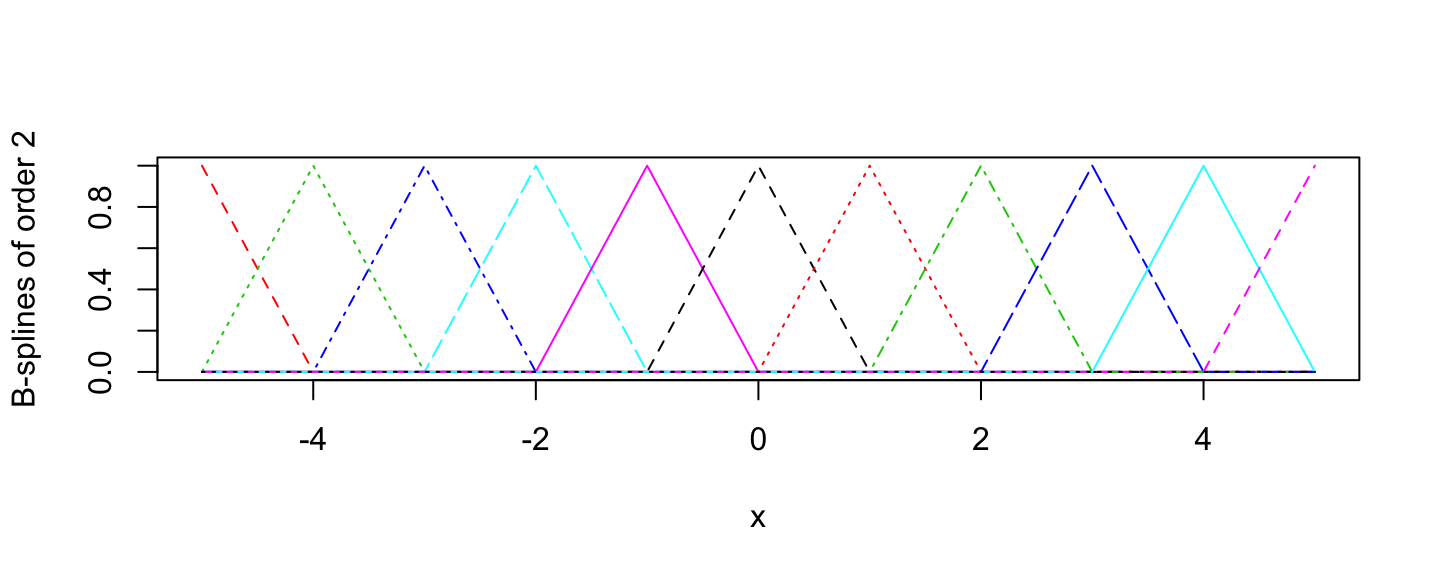# Overview

In this document, we discuss the implementation of splines in Stan. We start by providing a brief introduction to splines and then explain how they can be implemented in Stan. We also discuss a novel prior that alleviates some of the practical challenges of spline models.

# Introduction to Splines

Splines are continuous, piece-wise polynomial functions. B-splines or basis splines are the building blocks of spline functions: any spline function of a given degree can be expressed as a linear combination of B-splines of that degree. There are two parameters that uniquely define a family of B-spline functions: (i) the polynomial degree, $$p$$; and (ii) a non-decreasing sequence of knots, $$t_1, \ldots, t_q$$. The order of a spline family is defined as $$p+1$$. B-splines of order 1 ($$p=0$$) are a set of piece-wise constant functions: $B_{i,1}(x) := \left\{ \begin{matrix} 1, & \mathrm{if} \quad t_i \leq x < t_{i+1} \\ 0, & \mathrm{otherwise,} \end{matrix} \right.$ where $$B_{i,k}$$ denotes the $$i$$’th member of a family of B-splines of order $$k$$ (or equivalently of degree $$k-1$$). B-splines of higher orders are defined recursively: $B_{i,k}(x) := \omega_{i,k} B_{i,k-1}(x) + (1-\omega_{i+1, k}) B_{i+1,k-1}(x).$ where $\omega_{i,k} := \left\{ \begin{matrix} \frac{x - t_i}{t_{i+k-1} - t_i}, & \mathrm{if} \quad t_i \neq t_{i+k-1} \\ 0, & \mathrm{otherwise.} \end{matrix} \right.$ Thus, at a given point, $$x$$, a B-spline function of order $$k$$ is a linar combination of two B-splines of order $$k-1$$. To have well-defined B-splines of order $$k$$ covering the whole span of the knots, i.e., the interval $$[t_1, t_q)$$, the sequence of knots have to be extended as follows: $\mathrm{extended \ knots: } \ \underbrace{t_1,\ldots, t_1}_{k-1 \ \rm{ times}}, \underbrace{t_1, t_2, t_3, \ldots, t_q,}_{\rm original \ knot\ sequence} \underbrace{t_q, \ldots, t_q}_{k-1 \ \rm{ times}}.$ The recursive formulation described above is defined over the extended knots. If we do not extend the sequence of knots, we will not have B-spline functions covering the areas around the edges of the interval $$[t_1, t_q)$$. The second-order B-spline, $$B_{i,2}$$, has, in general, two linear pieces which join continuously at $$t_{i+1}$$ to form a piecewise linear function and vanishes outside the interval $$[t_i, t_{i+2})$$. A family of second-order B-splines for knots$$=\{-5,-4,-3,-2,-1,0,1,2,3,4,5\}$$ is shown below:As we can see, all the first-order B-splines, except the first and the last ones, consist of two linear pieces. Those two splines are defined around the edge of the interval, and because of the knot extension, have two equal knots. Similarly, B-splines of order $$k$$ consist of $$k$$ pieces of polynomials with degree $$k-1$$ (except those B-splines near the borders). These $$k$$ pieces are joined continuously at $$k-1$$ interior knots and moreover, are $$k-2$$ times differentiable. For instance, B-splines of order 4 have differenitals of orders 1 and 2; that is why the fourth-order B-splines (which consist of cubic polynomials) are widely used in practice. In the Figure below, we show a family of fourth-order B-splines: